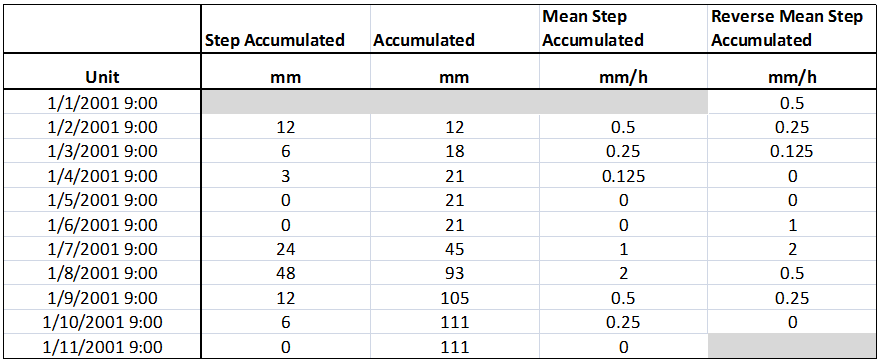What is a Time series?

A Time series is a sequence of data points (X, Y), measured typically at successive points in time spaced at uniform or non-uniform time intervals (X-Axis). An example of time series is the annual flow volume of a river at specific location.

Time series value (y-axis) types

In the DSS, the data can represented in different ways, which are called time series value types (e.g. Y-axis value types). These types are:

· Step accumulated: Data is accumulated for each time step and assigned to the next time step. Units can be mm.

· Accumulated: Data is accumulated for each time step and also all preceding time steps and assigned to the next time step.

· Mean step accumulated: Same as step accumulated but data is averaged across the time step and assigned to the next time step. Units can be mm/hour.

· Reverse Mean step accumulated: Same as Mean step accumulated but data is assigned to the present (actual) time step. Units can be mm/hour.

An example of the above types is given below:

Table 1: Time Series value type examples1) For Step Accumulated, Accumulated and Mean step Accumulated, the first time step should be empty as a value represents the period from previous to present (Actual) time step

2) For Reverse Mean Step Accumulated, the last time step should be empty as a value represents the period from present (actual) to the next time step

In addition to the above types, an ‘Instantaneous’ value type time series exists in the DSS. In this value type, data represent values at one precise instant at the given time. For example, the measured discharge or water level at gauge location is an instantaneous value.

Time series time (x-axis) types

In the DSS, the time data can represented in two ways which are called time series time axis types (i.e. X-axis types). These types are:

· Equidistant calendar: Data is equally spaced in time. An example of this is a daily rainfall time series data where the time step between data points is one day.

· Non-equidistant calendar: Data is not equally spaced in time. An example of this is a monthly rainfall time series data where the time step between data point is one month (so time step can be 28, 29, 30 or 31 days). The same applies to yearly data.